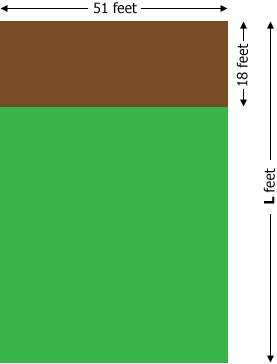SEARCH HOMEMath Central Quandaries & QueriesQuestion from Lucy, a student: Your boss says that his wife has put an 18 x 51 foot garden in along the whole back end of their back yard. He says that this has reduced the back-yard lawn area by 24%. What are the total dimensions of his back yard? What are the dimensions of the remaining lawn area? I found this question on purplemath(http://www.purplemath.com/modules/percntof3.htm). I still don't understand how to solve it. Can you walk me through the process please? Thank you in advance for taking the time to answer my question.Hi Lucy,I let L feet be the length of the entire yard.

The area of the garden is $18 \times 51 = 918$ square feet and you are told that this is $24 \%$ of the area of the entire back yard. Suppose that the area of the back yard is $x$ square feet then the garden area, 918 square feet, is $24 \%$ of $x.$ That is

$918 = 0.24 \times x.$

Solve for $x$ by dividing both side by 0.24 to get

$x = \frac{918}{0.24} = 3825 \mbox{ square feet.}$

From the diagram the area of the back yard is $51 \times L$ square feet and hence

$51 \times L = 3825 \mbox{ square feet.}$

This time solve for $L$ by dividing both sides by 51 to get

$L = \frac{3825}{51} = 75 \mbox{ feet.}$

I hope this helps,
PennyMath Central is supported by the University of Regina and The Pacific Institute for the Mathematical Sciences.